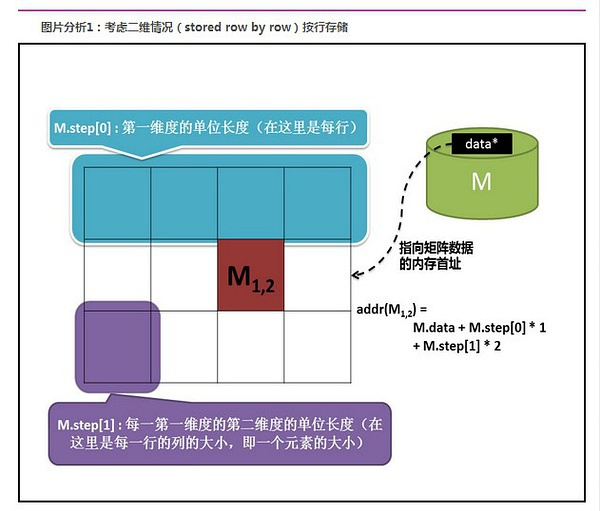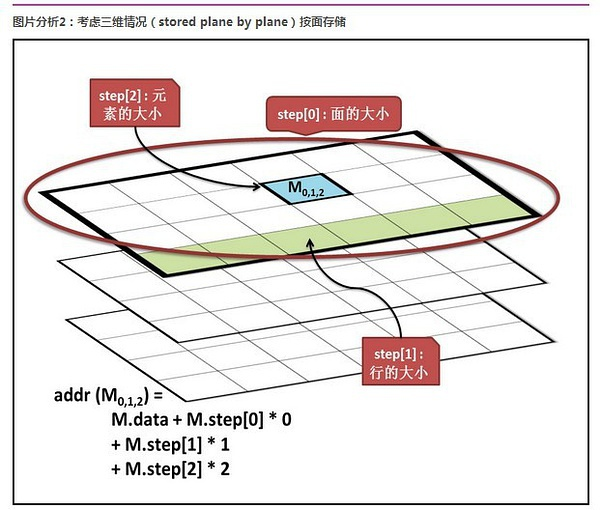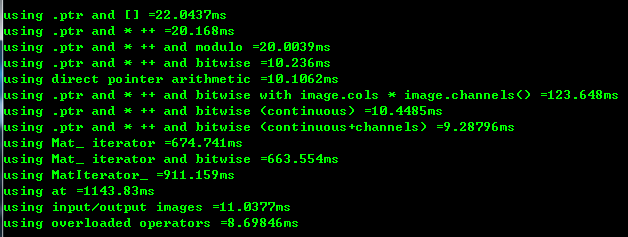• 一、数字图像存储概述数字图像存储时，我们存储...2、从OpenCV2开始，开始使用Mat类存储图像，具有以下优势：(1)图像的内存分配和释放由Mat类自动管理(2)Mat类由两部分数据组成：矩阵头(包含矩阵尺寸、存储方法、存...

一、数字图像存储概述
数字图像存储时，我们存储的是图像每个像素点的数值，对应的是一个数字矩阵。
二、Mat的存储
1、OpenCV1基于C接口定义的图像存储格式IplImage*，直接暴露内存，如果忘记释放内存，就会造成内存泄漏。
2、从OpenCV2开始，开始使用Mat类存储图像，具有以下优势：
(1)图像的内存分配和释放由Mat类自动管理
(2)Mat类由两部分数据组成：矩阵头(包含矩阵尺寸、存储方法、存储地址等)和一个指向存储所有像素值的矩阵(根据所选存储方法的不同，矩阵可以是不同的维数)的指针。Mat在进行赋值和拷贝时，只复制矩阵头，而不复制矩阵，提高效率。如果矩阵属于多个Mat对象，则通过引用计数来判断，当最后一个使用它的对象，则负责释放矩阵。
(3)可以使用clone和copyTo函数，不仅复制矩阵头还复制矩阵。
三、Mat创建
1、使用Mat构造函数
Mat test(2,2,CV_8UC3,Scalar(0,0,255));
2、使用Mat构造函数2
int sizes = {2,2,2};
Mat test(3,sizes,CV_8UC3,Scalar::all(0));
3、为已存在的IplImage指针创建信息头
Mat test(img);
4、利用create函数
Mat test;
test.create(4,4,CV_8UC2);
5、采用Matlab形式的初始化方式
(1)Mat me = Mat::eye(4,4,CV_64F);
(2)Mat mo = Mat::ones(2,2,CV_32F);
(3)Mat mz = Mat::zeros(3,3,CV_8UC1);
注：元素类型，即CV_[位数][带符号与否][类型前缀]C[通道数]
四、Mat中相关成员的意义
1、data
Mat对象中的一个指针，指向存放矩阵数据的内存(uchar* data)
2、dims
矩阵的维度，3*4的矩阵维度为2维，3*4*5的矩阵维度为3维
3、channels
矩阵通道，矩阵中的每一个矩阵元素拥有的值的个数，比如说 3 * 4 矩阵中一共 12 个元素，如果每个元素有三个值，那么就说这个矩阵是 3 通道的，即 channels = 3。常见的是一张彩色图片有红、绿、蓝三个通道。
4、depth
深度，即每一个像素的位数，也就是每个通道的位数。在opencv的Mat.depth()中得到的是一个0 – 6的数字，分别代表不同的位数：enum { CV_8U=0, CV_8S=1, CV_16U=2, CV_16S=3, CV_32S=4, CV_32F=5, CV_64F=6 }，可见 0和1都代表8位， 2和3都代表16位，4和5代表32位，6代表64位。
5、elemSize
矩阵中每个元素的大小，每个元素包含channels个通道。如果Mat中的数据的数据类型是CV_8U那么elemSize = 1；是CV_8UC3那么elemSize = 3，是CV_16UC2那么elemSize = 4。
6、elemSize1
矩阵中数据类型的大小，即elemSize/channels，也就是depth对应的位数。
7、step
是一个数组，定义了矩阵的布局，参考下图若矩阵有n维，则step数组大小为n
step[n-1] = elemSize(每个矩阵元素的数据大小)
step[n-2] = size(1维)*elemSize
step[n-3] = size(2维)*size(1维)*elemSize
...
step = size(n-1维)*size(n-2维)*...size(1维)*elemSize
8、step1
step1也是一个数组，为step/elemSize1，若矩阵有n维，则step1[n-1] = channels。
9、type
矩阵元素的类型，即创建Mat时传递的类型，例如CV_8UC3、CV_16UC2等。
OpenCV中Mat的列向量归一化
OpenCV中Mat的列向量归一化 http://blog.csdn.net/shaoxiaohu1/article/details/8287528 OpenCV中Mat的列向量归一化 标签: Ope ...
Opencv中Mat矩阵相乘——点乘、dot、mul运算详解
Opencv中Mat矩阵相乘——点乘.dot.mul运算详解 2016年09月02日 00:00:36 -牧野- 阅读数:59593 标签: Opencv矩阵相乘点乘dotmul 更多 个人分类: O ...
opencv中mat类介绍
The class Mat represents an n-dimensional dense numerical single-channel or multi-channel array. It ...
OpenCV中Mat操作clone&lpar;&rpar; 与copyto&lpar;&rpar;的区别
OpenCV中Mat操作clone() 与copyto()的区别 // Mat is basically a class with two data parts: the matrix header ...
OpenCV中Mat的属性
OpenCV中Mat的属性 最近在做一OpenCV的图像轮廓检验,但当用到霍夫变换时才发现对Mat的属性了解不足.Mat在OpenCV中的地位是及其重要的,因此有必要做一个总结. 大体上来说,Mat是 ...
OpenCV中Mat的基本用法：创建、复制
OpenCV中Mat的基本用法:创建.复制 一.Mat类的创建: 1.方法一: 通过读入一张图像,直接将其转换成Mat对象. Mat image = imread("test.jpg&quo ...
opencv中Mat与IplImage&comma;CVMat类型之间转换
opencv中对图像的处理是最基本的操作,一般的图像类型为IplImage类型,但是当我们对图像进行处理的时候,多数都是对像素矩阵进行处理,所以这三个类型之间的转换会对我们的工作带来便利. Mat类型 ...
opencv中Mat类型数据操作与遍历
Mat作为opencv中一种数据类型常常用来存储图像,相对与以前的IplImgae类型来说,Mat类型省去了人工的对内存的分配与释放,转而自动分配释放.Mat Class主要包括两部个数据部分:一个是 ...
OpenCV中Mat总结
一.数字图像存储概述 数字图像存储时,我们存储的是图像每个像素点的数值,对应的是一个数字矩阵. 二.Mat的存储 1.OpenCV1基于C接口定义的图像存储格式IplImage*,直接暴露内存,如果忘 ...
随机推荐
win7安装virtualbox遇到的问题
今天用台式机的时候想装个virtualbox跑centos做测试用,结果centos始终装不上,vbox一直提示无法开启任务.重装vbox,以及手动点击安装xxx.inf文件,都不行. 以前用的win ...
QQ游戏&lowbar;相关
【MySQL】查看MySQL配置文件路径及相关配置
(1)关于配置文件路径 有时候,我发现虽然尝试修改了配置文件的一些变量,但是并没有生效.后来才发现原来是因为修改的文件并非MySQL服务器读取的配置文件. 如果不清楚MySQL当前使用的配置文件路径, ...
Windows开启Telnet
为了防止无良网站的爬虫抓取文章,特此标识,转载请注明文章出处.LaplaceDemon/SJQ. http://www.cnblogs.com/shijiaqi1066/p/4301513.html ...
4D(DRG、DLG、DOM、DEM)数据 概念
抱歉原文链接未知 Technorati 标签: DRG,DLG,DOM,DEM 以下有不同的说法,但是意思都很相近. 一. DOM (数字正射影像图):利用数字高程模型对扫描处理的数字化的航空相片.遥 ...
ieee trans pami latex模板
https://www.computer.org/cms/Computer.org/transactions/templates/ https://www.computer.org/web/tpami ...
phpdocumentor安装和使用总结
为了解决一校友在安装和使用phpDocumentor过程中遇到的问题,自己闲时也折腾了一下这个东西,总结见下: 一.定义: 自己刚听到这个词时还不知道这个是什么东西,干啥用的,就去百度了一下,说道: ...
pygame&period;error&colon; Couldn&&num;39&semi;t open images&sol;ship&period;bmp
在这本书中的的项目里有如下代码:  Python Code  123456789101112131415   import py ...
SpringBoot简介及第一个应用
一.Spring时代变换 1. Spring1.x 时代 Spring初代都是通过xml文件配置bean,随着项目的不断扩大,繁琐的xml配置,混乱的依赖关系,难用的bean装配方式,由此衍生了spr ...

展开全文• //整理创建Mat的方法 //1. 使用Mat()构造函数构建 /** @overload @param rows Number of rows in a 2D array. 二维数组的行数，又图像的高度 @param cols Number of columns in a 2D array. 二维数组的列数，又...
//整理创建Mat的方法
//1. 使用Mat()构造函数构建
@param rows Number of rows in a 2D array.    二维数组的行数，又图像的高度
@param cols Number of columns in a 2D array. 二维数组的列数，又图像的宽度
@param type Array type. Use CV_8UC1, ..., CV_64FC4 to create 1-4 channel matrices, or
CV_8UC(n), ..., CV_64FC(n) to create multi-channel (up to CV_CN_MAX channels) matrices. 数组的类型，又图片的类型
*/
//Mat(int rows, int cols, int type);
Mat m(120, 120, CV_8UC3); //创建120×120的三通道图像，图像的像素值随机生成
randu(m, Scalar::all(0), Scalar::all(255));//也可以自己指定随机值的期望范围
Mat m2(120, 120, CV_8UC3, Scalar(0, 255, 0));//创建120×120的三通道图像，指定图像的像素值

//2. 采用Matlab式初始化
Mat eyeImg = Mat::eye(4, 4, CV_8UC3);//创建对角线为1，其余为0的图像
Mat oneImg = Mat::ones(4, 4, CV_8UC3);//创建像素值全为1的图像
Mat zeroImg = Mat::zeros(4, 4, CV_8UC3);//创建像素值全为0的图像

//3. 从已有的图像处拷贝
Mat cloneImg = eyeImg.clone();
Mat copyImg;
eyeImg.copyTo(copyImg);

展开全文1.1 Mat对象的概念
1.2 Mat读取图像基本信息
Mat对象除了存储图像的像素数据以外，还包括图像的其他属性，具体为宽、高、类型、维度、大小、深度等。具体获取API如下：
int width = src.cols();//宽
int height = src.rows();//高
int dims = src.dims();//维度
int channels = src.channels();//通道数
int depth = src.depth();//深度
int type = src.type();//类型信息
{
return retVal;
}
这里重点关注通道数、图像深度与图像类型、Op en C V 加载的Mat 类型图
像对象。 1.2.1 通道数
常见的通道数目有1 、3 , 4 ，分别对应于单通道、三通道、四通道，其中四通道
中通常会有透明通道的数据。 1.2.2 图像深度
图像深度表示每个通道灰度值所占的大小， 图像深度与类
型密切相关。Open CV 中常见的几种图像深度具体如下表所示。
图像深度Java 中对应的数据类型图像深度Java 中对应的数据类型
其中， U 表示无符号整型、S 表示符号整型、F 表示浮点数， 这些类型在CvType 中可以自己查看。
1.3 Mat对象的新建
创建Mat 对象的方法有很多种，其中最常见的有如下几种。
1 ）通过create 方法实现Mat 对象的创建，相关的代码如下：
Mat ml = new Mat();
ml.create(new Size(3, 3), CvType.CV_ 8UC3);
Mat m2 = new Mat();
m2.create(3, 3, CvType.CV_8UC3); 图2 -2
上述代码表示创建两个Mat 对象ml 与m2 ，其中m l 与m2 的大小都是3 × 3 、类型是三通道8 位的无符号字符型。
2 ）通过ones 、eye 、zeros 方法实现初始化创建，相关代码如下：
Mat m3 = Mat.eye(3, 3,CvType.CV 8UC3);
Mat m4 = Mat.eye(new Size(3, 3),CvType.CV 8UC3);
Mat m5 = Mat.zeros(new Size(3, 3) , CvType.CV 8UC3);
Mat m6 = Mat.ones(new Size(3, 3), CvType.CV_8UC3);
上述代码创建了m3 , m4 、m5 , m6 四个Mat 对象，基于这种初始化方式来得到Mat对象是OpenCV 借鉴了Matlab 中eye 、zeros 、ones 三个函数实现的。
3 ）此外还可以先定义Mat ，然后通过setTo 的方法实现初始化，相关代码如下：
Mat m7 =new Mat(3, 3, CvType.CV 8UC3);
m7.setTo(new Scalar(255, 255, 255));
此方法与第一种方法有点类似，唯一不同的是，第一种方法通过create 初始化的时候并没有指定颜色值。在OpenCV 中，颜色向量通常用Scalar 表示， 这里Sca lar ( 255 ,255’ 255 ）表示白色。
4 ）通过Mat 的copyTo （）与clone 方法实现对象的创建， Mat 中的克隆与拷贝方法会复制一份完全相同的数据以创建一个新Mat 对，克隆相关代码如下：
Mat m8 =new Mat(500, 500, CvType.CV 8UC3);
m8.setTo(new Scalar(l27, 127, 127));
Mat cmat =image.clone();
拷贝的相关代码如下：
Mat m8 =new Mat(500, 500, CvType.CV 8UC3);
m8.setTo(new Scalar(l27, 127, 127));
Mat result = new Mat();
m8.copyTo(result);
1.4 Mat对象保存
创建好的Mat 对象经过一系列的操作之后，就可以通过OpenCV4Android 的imwrite函数直接将对象保存为图像，相关的演示代码如下：
／／ 创建Mat 对象并保存
Ma t image = new Mat(SOO, 500, CvType.CV_8UC3);
image.setTo(new Scalar(l27 , 127, 127));
ImageSelectUtils.sav eimage (image );
其中， 500 表示图像的宽度与高度，最后一个参数声明图像是RGB 彩色三通道图像、
每个通道都是8 位，第二行代码是指定图像的每个像素点、每个通道的灰度值为127 。
第三行代码是使用imwrite 将图像保存到手机中的指定目录下， save Image 方法的相关代
码如下：
File fileDir = new File(Environment.getExternalStor agePublicDirectory(
Env ironment.DIRECTORY P 工CTURES), “mybook”);
if ( ! fileDir. exists ( ) ) {
fileDir.mkdirs ();
String name= String. v alueOf(System.currentTimeMillis()) +" book.jpg”;
File tempFile =new File (file Dir.getAbsoluteFile()+File.separator, name);
Imgcodecs.imwrite (tempFile . getAbsolutePath(), image);
上面的前几行代码是创建目录与文件路径，最后一行代码通过imwrite 来实现文件
的保存， 保存图像的格式取决于文件路径为图像指定的扩展名类型。
展开全文• ## opencv 获取Mat像素值

千次阅读 2016-03-11 09:21:51
opencv 访问Mat中每个像素的值 转自：http://blog.csdn.net/xiaowei_cqu/article/details/19839019 方法零：.ptr和[]操作符 Mat最直接的访问方法是通过.ptr [cpp] view plain copy   ...
opencv 访问Mat中每个像素的值
转自：http://blog.csdn.net/xiaowei_cqu/article/details/19839019

方法零：.ptr和[]操作符

Mat最直接的访问方法是通过.ptr<>函数得到一行的指针，并用[]操作符访问某一列的像素值。

[cpp]
view plain
copy// using .ptr and []   void colorReduce0(cv::Mat &image, int div=64) {         int nr= image.rows; // number of rows         int nc= image.cols * image.channels(); // total number of elements per line         for (int j=0; j<nr; j++) {             uchar* data= image.ptr<uchar>(j);             for (int i=0; i<nc; i++) {                     data[i]= data[i]/div*div + div/2;               }                           }   }

方法一：.ptr和指针操作
除了[]操作符，我们可以移动指针*++的组合方法访问某一行中所有像素的值。

[cpp]
view plain
copy// using .ptr and * ++    void colorReduce1(cv::Mat &image, int div=64) {         int nr= image.rows; // number of rows         int nc= image.cols * image.channels(); // total number of elements per line         for (int j=0; j<nr; j++) {             uchar* data= image.ptr<uchar>(j);             for (int i=0; i<nc; i++) {                    *data++= *data/div*div + div/2;               } // end of row                          }   }

方法二：.ptr、指针操作和取模运算
方法二和方法一的访问方式相同，不同的是color reduce用模运算代替整数除法

[cpp]
view plain
copy// using .ptr and * ++ and modulo   void colorReduce2(cv::Mat &image, int div=64) {         int nr= image.rows; // number of rows         int nc= image.cols * image.channels(); // total number of elements per line         for (int j=0; j<nr; j++) {             uchar* data= image.ptr<uchar>(j);             for (int i=0; i<nc; i++) {                     int v= *data;                     *data++= v - v%div + div/2;               } // end of row                          }   }

方法三：.ptr、指针运算和位运算
由于进行量化的单元div通常是2的整次方，因此所有的乘法和除法都可以用位运算表示。

[cpp]
view plain
copy// using .ptr and * ++ and bitwise   void colorReduce3(cv::Mat &image, int div=64) {         int nr= image.rows; // number of rows         int nc= image.cols * image.channels(); // total number of elements per line         int n= static_cast<int>(log(static_cast<double>(div))/log(2.0));         // mask used to round the pixel value         uchar mask= 0xFF<<n; // e.g. for div=16, mask= 0xF0         for (int j=0; j<nr; j++) {             uchar* data= image.ptr<uchar>(j);             for (int i=0; i<nc; i++) {               *data++= *data&mask + div/2;               } // end of row                          }   }

方法四：指针运算
方法四和方法三量化处理的方法相同，不同的是用指针运算代替*++操作。

[cpp]
view plain
copy// direct pointer arithmetic   void colorReduce4(cv::Mat &image, int div=64) {         int nr= image.rows; // number of rows         int nc= image.cols * image.channels(); // total number of elements per line         int n= static_cast<int>(log(static_cast<double>(div))/log(2.0));         int step= image.step; // effective width         // mask used to round the pixel value         uchar mask= 0xFF<<n; // e.g. for div=16, mask= 0xF0         // get the pointer to the image buffer         uchar *data= image.data;         for (int j=0; j<nr; j++) {             for (int i=0; i<nc; i++) {               *(data+i)= *data&mask + div/2;               } // end of row                                data+= step;  // next line         }   }

方法五：.ptr、*++、位运算以及image.cols * image.channels()
这种方法就是没有计算nc，基本是个充数的方法。

[cpp]
view plain
copy// using .ptr and * ++ and bitwise with image.cols * image.channels()   void colorReduce5(cv::Mat &image, int div=64) {         int nr= image.rows; // number of rows         int n= static_cast<int>(log(static_cast<double>(div))/log(2.0));         // mask used to round the pixel value         uchar mask= 0xFF<<n; // e.g. for div=16, mask= 0xF0         for (int j=0; j<nr; j++) {             uchar* data= image.ptr<uchar>(j);             for (int i=0; i<image.cols * image.channels(); i++) {               *data++= *data&mask + div/2;               } // end of row                          }   }

方法六：连续图像
Mat提供了isContinuous()函数用来查看Mat在内存中是不是连续存储，如果是则图片被存储在一行中。

[cpp]
view plain
copy// using .ptr and * ++ and bitwise (continuous)   void colorReduce6(cv::Mat &image, int div=64) {         int nr= image.rows; // number of rows         int nc= image.cols * image.channels(); // total number of elements per line         if (image.isContinuous())  {             // then no padded pixels             nc= nc*nr;              nr= 1;  // it is now a 1D array          }         int n= static_cast<int>(log(static_cast<double>(div))/log(2.0));         // mask used to round the pixel value         uchar mask= 0xFF<<n; // e.g. for div=16, mask= 0xF0         for (int j=0; j<nr; j++) {             uchar* data= image.ptr<uchar>(j);             for (int i=0; i<nc; i++) {               *data++= *data&mask + div/2;               } // end of row                          }   }

方法七：continuous+channels
与方法六基本相同，也是充数的。

[cpp]
view plain
copy// using .ptr and * ++ and bitwise (continuous+channels)   void colorReduce7(cv::Mat &image, int div=64) {         int nr= image.rows; // number of rows         int nc= image.cols ; // number of columns         if (image.isContinuous())  {             // then no padded pixels             nc= nc*nr;              nr= 1;  // it is now a 1D array          }         int n= static_cast<int>(log(static_cast<double>(div))/log(2.0));         // mask used to round the pixel value         uchar mask= 0xFF<<n; // e.g. for div=16, mask= 0xF0         for (int j=0; j<nr; j++) {             uchar* data= image.ptr<uchar>(j);             for (int i=0; i<nc; i++) {               *data++= *data&mask + div/2;               *data++= *data&mask + div/2;               *data++= *data&mask + div/2;               } // end of row                          }   }

方法八：Mat _iterator
真正有区别的方法来啦，用Mat提供的迭代器代替前面的[]操作符或指针，血统纯正的官方方法~

[cpp]
view plain
copy// using Mat_ iterator    void colorReduce8(cv::Mat &image, int div=64) {         // get iterators         cv::Mat_<cv::Vec3b>::iterator it= image.begin<cv::Vec3b>();         cv::Mat_<cv::Vec3b>::iterator itend= image.end<cv::Vec3b>();         for ( ; it!= itend; ++it) {           (*it)= (*it)/div*div + div/2;           (*it)= (*it)/div*div + div/2;           (*it)= (*it)/div*div + div/2;         }   }

方法九：Mat_ iterator 和位运算
把方法八中的乘除法换成位运算。

[cpp]
view plain
copy// using Mat_ iterator and bitwise   void colorReduce9(cv::Mat &image, int div=64) {         // div must be a power of 2         int n= static_cast<int>(log(static_cast<double>(div))/log(2.0));         // mask used to round the pixel value         uchar mask= 0xFF<<n; // e.g. for div=16, mask= 0xF0         // get iterators         cv::Mat_<cv::Vec3b>::iterator it= image.begin<cv::Vec3b>();         cv::Mat_<cv::Vec3b>::iterator itend= image.end<cv::Vec3b>();         for ( ; it!= itend; ++it) {           (*it)= (*it)&mask + div/2;           (*it)= (*it)&mask + div/2;           (*it)= (*it)&mask + div/2;         }   }

方法十：MatIterator_
和方法八基本相同。

[cpp]
view plain
copy// using MatIterator_    void colorReduce10(cv::Mat &image, int div=64) {         cv::Mat_<cv::Vec3b> cimage= image;         cv::Mat_<cv::Vec3b>::iterator it=cimage.begin();         cv::Mat_<cv::Vec3b>::iterator itend=cimage.end();         for ( ; it!= itend; it++) {            (*it)= (*it)/div*div + div/2;           (*it)= (*it)/div*div + div/2;           (*it)= (*it)/div*div + div/2;         }   }

方法十一：图像坐标

[cpp]
view plain
copy// using (j,i)   void colorReduce11(cv::Mat &image, int div=64) {         int nr= image.rows; // number of rows         int nc= image.cols; // number of columns         for (int j=0; j<nr; j++) {             for (int i=0; i<nc; i++) {                     image.at<cv::Vec3b>(j,i)=     image.at<cv::Vec3b>(j,i)/div*div + div/2;                     image.at<cv::Vec3b>(j,i)=     image.at<cv::Vec3b>(j,i)/div*div + div/2;                     image.at<cv::Vec3b>(j,i)=     image.at<cv::Vec3b>(j,i)/div*div + div/2;               } // end of row                          }   }

方法十二：创建输出图像
之前的方法都是直接修改原图，方法十二新建了输出图像，主要用于后面的时间对比。

[cpp]
view plain
copy// with input/ouput images   void colorReduce12(const cv::Mat &image, // input image                     cv::Mat &result,      // output image                    int div=64) {         int nr= image.rows; // number of rows         int nc= image.cols ; // number of columns         // allocate output image if necessary         result.create(image.rows,image.cols,image.type());         // created images have no padded pixels         nc= nc*nr;          nr= 1;  // it is now a 1D array         int n= static_cast<int>(log(static_cast<double>(div))/log(2.0));         // mask used to round the pixel value         uchar mask= 0xFF<<n; // e.g. for div=16, mask= 0xF0         for (int j=0; j<nr; j++) {             uchar* data= result.ptr<uchar>(j);             const uchar* idata= image.ptr<uchar>(j);             for (int i=0; i<nc; i++) {               *data++= (*idata++)&mask + div/2;               *data++= (*idata++)&mask + div/2;               *data++= (*idata++)&mask + div/2;             } // end of row                          }   }

方法十三：重载操作符
Mat重载了+&等操作符，可以直接将两个Scalar(B,G,R)数据进行位运算和数学运算。

[cpp]
view plain
copy时间对比
通过迭代二十次取平均时间，得到每种方法是运算时间如下。可以看到，指针*++访问和位运算是最快的方法；而不断的计算image.cols*image.channles()花费了大量重复的时间；另外迭代器访问虽然安全，但性能远低于指针运算；通过图像坐标(j,i)访问时最慢的，使用重载操作符直接运算效率最高。

展开全文• 原创 OpenCv2 学习笔记(1) Mat创建、复制、释放 ...
• ## opencv创建Mat对象

千次阅读 2019-03-31 17:36:04
opencv中引入了Mat类。 Mat类是一个非常优秀的图像类，它同时也是一个通用的矩阵类，可以用来创建和操作多维矩阵。Mat类里面定义了许多构造函数，可以很方便地根据要求创建Mat对象。 常使用的构造函数有： 1：...
• 获取通道channels=1，Mat元素类型为CV_32SC1的元素的值 使用ptr指针 ...opencv2\opencv.hpp> #include<iostream> using namespace cv; using namespace std; int main() { Mat mat(5...
• ## opencv 取Mat元素的值

万次阅读 2016-08-30 10:50:04
获取通道channels=1，Mat元素类型为CV_32SC1的元素的值 1）使用ptr指针如 mat.ptr(i)+j) 形式 注意： （1）元素类型是什么类型就用什么类型的指针，如CV_32SC1为32位有符号1通道矩阵，用uchar*指针，CV_32FC1为32...
• 背景：代码的原理是opencv连接摄像头，捕获到帧数据mat（矩阵），将其转成byte数组然后进行base64编码，把数据与帧的其他信息（宽、高、通道数、时间戳）写入到json对象中，Kafka把json对象以字符串是方式发送出去；...python java kafka ceph
• 特征存取是机器学习过程中的...我打算使用opencvMat进行数据的保存，那么Mat如何构造，如何保存，如何读取呢？ Mat的构造 如下是创建一个Mat的方式，Size里边先填列数，再填行数！！这点和一般习惯有些不同，要记住哦
• 今天读取opencv中的Mat数组，已经被打败，真的是类型不对就读不出内容啊，真的要崩溃，故特此记录一下。 1、首先了解一下Mat中对应的各个类型 在opencv中像素点的数据类型能找到对应类似char，int，float,double的，...
• 先下载delphi opencv套件：... 打开opencv_classes工程， 增加如下 两个函数导出，用于mat->IplImage, IplImage->mat的转换， 因为delphi opencv这个套件里面的ocvView显示用的是IplImage。 ICLASS_API IplImage __stdelphi
• ## OpenCV的Mat基本用法

千次阅读 2015-03-05 10:31:58
Mat image(240, 320, CV8UC3); 第一个参数是rows，该矩阵的行数；第二个参数是cols，该矩阵的列数；第三个参数是该矩阵元素的类型。这句话表示创建一个大小为240×320的矩阵，里面的元素为8位unsigned型，通道数...
• Mat类表示的图像进行ROI操作有两种方法 1. 使用拷贝构造函数Mat(constMat&amp;amp; m, const Rect&amp;amp; roi )，矩形roi指定了兴趣区 Mat src = imread(“xx.jpg”); Mat srcROI( src, Rect(0,0,src...
• C++读写txt文件方式以及基于opencvMat数据类型读写txt文件
• cv::Mat image = cv::Mat(400, 600, CV_8UC1); //宽400，长600 uchar * data00 = image.ptr<uchar>(0); uchar * data10 = image.ptr<uchar>(1); uchar * data01 = image.ptr<uchar>(0); 1...
• 2018.10.8更新 不过最新的学习发现，如果CV::MAT中的数据是连续的，也可以把数据用memcpy拷贝到对应的data指针指向的内存中。不过需要注意的是如果不连续，这样做是不可以的。...inline Mat::Mat(int _ro...
• 结论是： Mat.at(i,j)的方法是很慢的，最好不要用这种方法来。而涉及到指针的方法效果往往比较好！（ O(∩_∩)O哈哈~这个不是作者说的哈，我说的） 在《OpenCV 2 Computer Vision Application Programming ...c++ 图片
• #include "stdafx.h" #include #include #include using namespace std; using namespace cv; int _tmain(int argc, _TCHAR* argv[]) { //创建一个用1+3j填充的 7 x 7 复...Mat M(7, 7, CV_32FC2,数据结构 include string c
• cv::Mat_<float> A=(Mat_<float>(3,2)<<1,7,2,3,4,5); cv::Mat_<float> B=(Mat_<float>(3,2)<<1,7,2,3,4,5); Mat C,D; vconcat(A,B,C); hconcat(A,B,D); cout<<C<<...
• OpenCV3图像处理】Mat中元素的获取与赋值 （ 对比.at&lt;&gt;()函数 和 .ptr&lt;&gt;()函数） 2017年04月12日 10:08:55 阅读数：7542 标签： opencvopencv3 更多 个人分类： Opencv 所属...
• 准备资料 Ubuntu16.04或者其他版本 Python2.x或者Python3.x boost_1_69_0或者其他版本 // ... OpenCV3.4.3或者其他版本 // https://www.opencv.org/releases.html pyboostcvconver...C++转python C/C++ python
• 现使用opencv对图像Mat类型读写txt文件进行汇总~ 首先将一幅图像写入txt文件中（实例1）： //#include //#include #include<opencv2\opencv.hpp> #include<core/core.hpp> #include...txt
• Mat img = image.clone(); if (img.channels() &gt; 1) { cvtColor(img, img, CV_RGB2GRAY); } Mat out(img.size(), img.type(), Scalar(255));//全白图 ...
• OpenCV Mat 存 取 共享内存 例子 ...# 重新开一个窗口，执行 opencv_mat_from_mem 可以将共享内存中的视频帧(mat)数据，放入到新建mat对象中，并转存到 I/O 磁盘上面 ./opencv_mat_from_mem 在操作系C++
• 我记得开始接触OpenCV就是因为一个算法里面需要2维动态数组，那时候看core这部分也算是走马观花吧，随着使用的增多，对Mat这个结构越来越喜爱，也觉得有必要温故而知新，于是这次再看看MatMat最大的优势跟STL很......